﻿ 新型中性线谐波电流抑制器

# 新型中性线谐波电流抑制器New Harmonic Current Suppressor

Abstract: This article is based on the improvement of the existing neutral-line harmonic filters. It is pro-posed that the conventional zig-zag transformer cannot suppress the zero-sequence harmonic current generated by unbalanced grid voltage, and the neutral line voltage offset problem that is easily caused by a simple neutral-line series filter is proposed. A novel neutral-line harmonic current suppression method combining a zero-sequence filter and a series-neutral-line filter is proposed. This method combines the zero-sequence filter based on zigzag transformer and the series neutral filter, which not only solves the problem of filtering the third harmonic, but also plays a good role in suppressing the midline zero-sequence current, and has little effect on the load.

1. 引言

2. 滤波器结构

2.1. 零序滤波器

2.2. 串联中线滤波器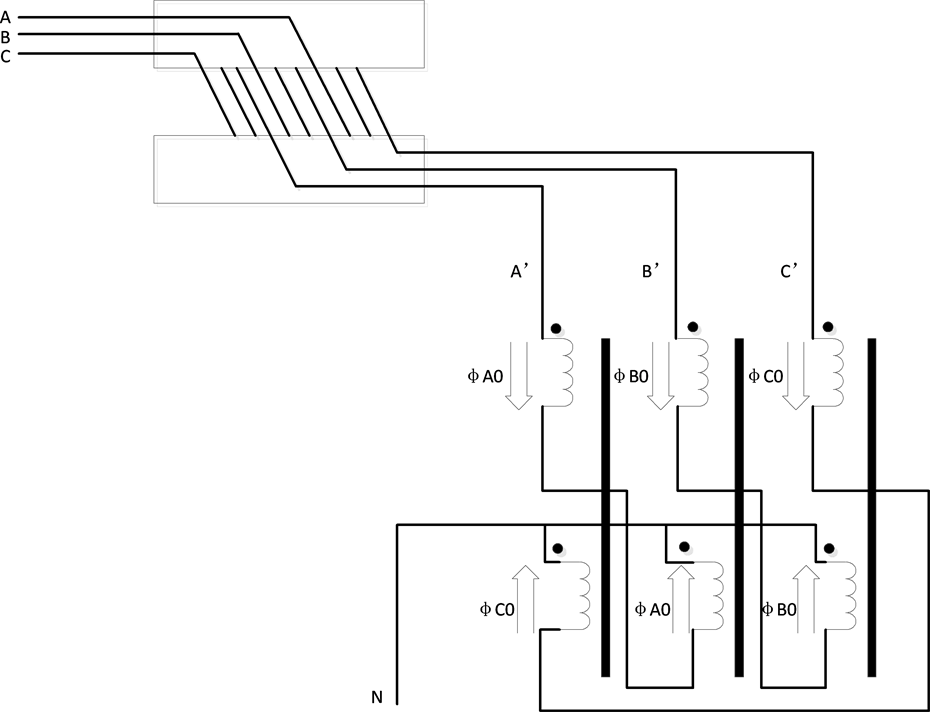Figure 1. Structure of Zero-sequence filter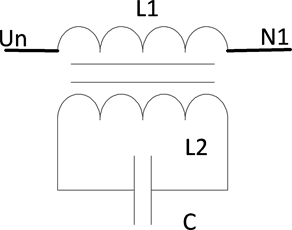Figure 2. Structure of Serial midline filter

${Z}_{f}=\frac{{X}_{L1}{X}_{C}}{{X}_{L1}+{X}_{L2}+2{X}_{M}+{X}_{C}}$

3. 等效电路分析

3.1. 零序等效电路

${i}_{sn}=\frac{{Z}_{zn}}{{Z}_{sn}+3{Z}_{f}+{Z}_{s}+{Z}_{z}+{Z}_{zn}}{i}_{s0}+\frac{1}{{Z}_{sn}+3{Z}_{f}+{Z}_{s}+{Z}_{z}+{Z}_{zn}}{u}_{s0}$

${i}_{zn}=\frac{{Z}_{sn}+3{Z}_{f}+{Z}_{s}+{Z}_{z}}{{Z}_{sn}+3{Z}_{f}+{Z}_{s}+{Z}_{z}+{Z}_{zn}}{i}_{s0}-\frac{1}{{Z}_{sn}+3{Z}_{f}+{Z}_{s}+{Z}_{z}+{Z}_{zn}}{u}_{s0}$

$\frac{{Z}_{sn}+3{Z}_{f}+{Z}_{s}+{Z}_{z}}{{Z}_{sn}+3{Z}_{f}+{Z}_{s}+{Z}_{z}+{Z}_{zn}}{i}_{s0}\gg \frac{{Z}_{zn}}{{Z}_{sn}+3{Z}_{f}+{Z}_{s}+{Z}_{z}+{Z}_{zn}}{i}_{s0}$

${u}_{zn}=\frac{\left({Z}_{sn}+3{Z}_{f}+{Z}_{s}+{Z}_{z}\right)\left({Z}_{zn}\right)}{{Z}_{sn}+3{Z}_{f}+{Z}_{s}+{Z}_{z}+{Z}_{zn}}{i}_{s0}-\frac{{Z}_{zn}}{{Z}_{sn}+3{Z}_{f}+{Z}_{s}+{Z}_{z}+{Z}_{zn}}{u}_{s0}$

$\frac{\left({Z}_{sn}+3{Z}_{f}+{Z}_{s}+{Z}_{z}\right)\left({Z}_{zn}\right)}{{Z}_{sn}+3{Z}_{f}+{Z}_{s}+{Z}_{z}+{Z}_{zn}}\approx {Z}_{zn}$

${u}_{zn}=\frac{\left({Z}_{sn}+3{Z}_{f}\right)\left({Z}_{zn}\right)}{{Z}_{sn}+3{Z}_{f}+{Z}_{s}+{Z}_{z}+{Z}_{zn}}{i}_{s0}+\frac{{Z}_{sn}+3{Z}_{f}}{{Z}_{sn}+3{Z}_{f}+{Z}_{s}+{Z}_{z}+{Z}_{zn}}{u}_{s0}$Figure 3. Zero sequence equivalent circuit

3.2. 三次谐波等效电路

${i}_{sn}=\frac{{Z}_{zn}}{{Z}_{sn}+{Z}_{f}+{Z}_{s}+{Z}_{z}+{Z}_{zn}}{i}_{3s}+\frac{1}{{Z}_{sn}+{Z}_{f}+{Z}_{s}+{Z}_{z}+{Z}_{zn}}{u}_{3s}$

4. 仿真分析

4.2. 仿真结果分析Figure 4. Third harmonic equivalent circuit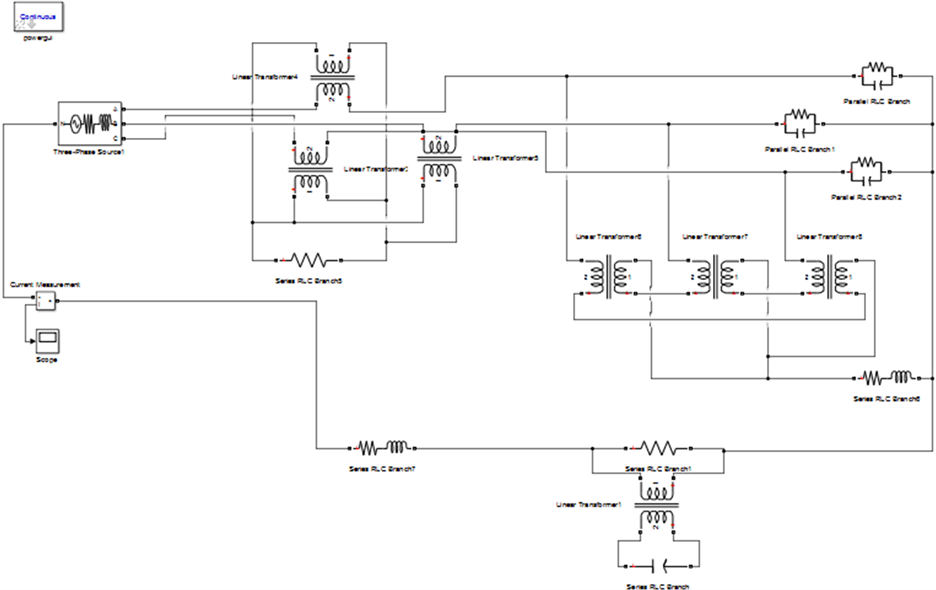Figure 5. Neutral harmonic current suppressor simulation model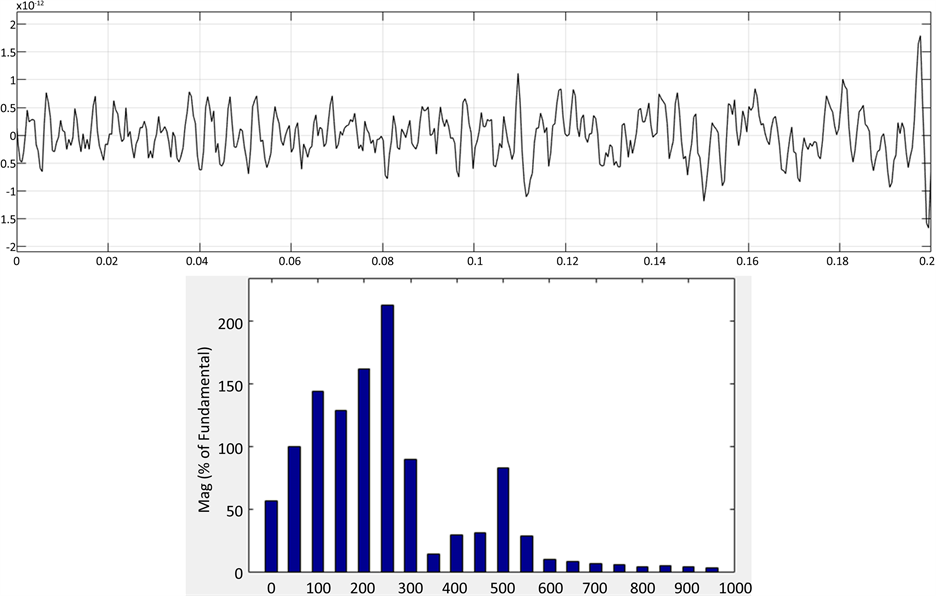Figure 6. System simulation waveform and FFT analysis without any filtering device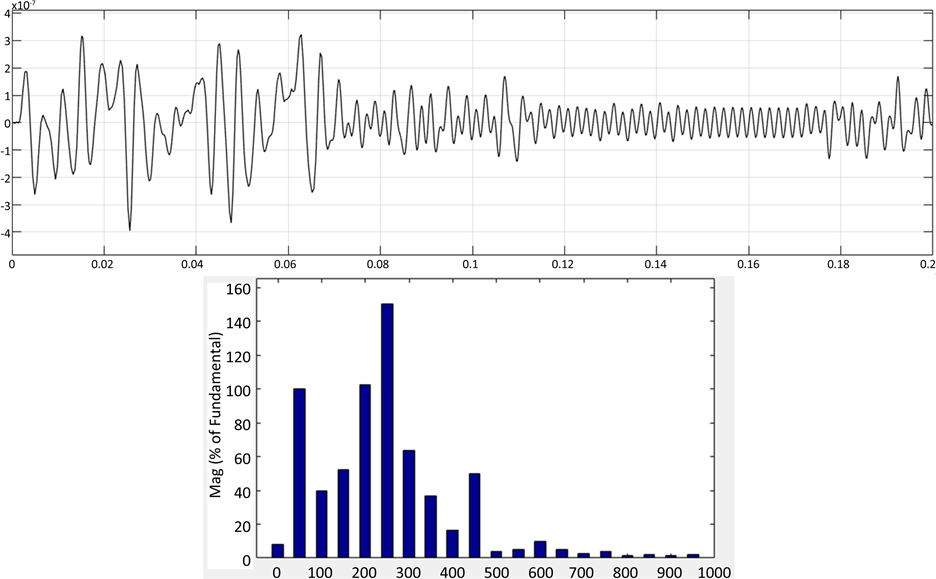Figure 7. System simulation waveform and FFT analysis only with zero-sequence filterFigure 8. Neutral harmonic current suppressor simulation waveform and FFT analysis

5. 结论

 王轩, 朱东起, 姜新建. 三相四线制下串联混合滤波器的研究[J]. 电力系统自动化, 2004, 28(3): 45-48.

 王轩, 朱东起, 姜新建. 治理三相四线制配电网谐波电流的新技术[J]. 电力系统自动化, 2004, 28(15): 44-47.

 孙建军, 查晓明, 丁凯. 一种低耐压中性线谐波治理电路原理及实现[J]. 电力系统自动化, 2003, 27(18): 34-37.

 Dahono, P.A. and Widjaya, R.E. (1997) A Practical Approach to Minimize the Zero Sequence Current Harmonics in Power Distribution Systems. IEEE Proceedings of Power Conversion Conference, Nagaoka, 6 August 1997, 683-686.
https://doi.org/10.1109/PCCON.1997.638278

 庞庆, 张云建, 占江山. 基于桥式逆变器中性线谐波电流治理装置的研究[J]. 电力电子技术, 2007, 41(11): 12-15.

 Zahira, R. and Fathima, A.P. (2012) A Technical Survey on Control Strategies of Active Filter for Harmonic Suppression. Procedia Engineering, 30, 686-693.
https://doi.org/10.1016/j.proeng.2012.01.915

 Khera, P.P. (1990) Application of Zig Zag Transformers for Reducing Harmonics in the Neutral Conductor of Low Voltage Distribution System. IEEE IAS, No. 2, 1092-1096.

Top